# GMAT Math : DSQ: Calculating the length of the side of an equilateral triangle

## Example Questions

2 Next →

### Example Question #11 : Equilateral Triangles

Given equilateral triangle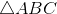and right triangle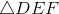, which, if either, is longer,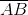or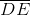?

Statement 1: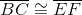Statement 2: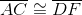BOTH statements TOGETHER are sufficient to answer the question, but NEITHER statement ALONE is sufficient to answer the question.

BOTH statements TOGETHER are insufficient to answer the question.

Statement 1 ALONE is sufficient to answer the question, but Statement 2 ALONE is NOT sufficient to answer the question.

Statement 2 ALONE is sufficient to answer the question, but Statement 1 ALONE is NOT sufficient to answer the question.

EITHER statement ALONE is sufficient to answer the question.

BOTH statements TOGETHER are sufficient to answer the question, but NEITHER statement ALONE is sufficient to answer the question.

Explanation:

Assume Statement 1 alone. Since all three sides ofare congruent - specifically,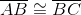- and, it follows by transitivity that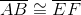. However, no information is given as to whetherhas length greater than, equal to, or less than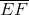, so it cannot be determined which ofand, if either, is the longer. By a similar argument, Statement 2 yields insufficient information.

Now assume both statements are true.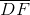andare each congruent to one of the congruent sides of equilateraland are therefore congruent to each other. However, the hypotenuse of a right triangle must be longer than both legs, so the hypotenuse ofisis also longer than any segment congruent to one of the legs, which includes all three sides of- specificially,is longer than.

### Example Question #12 : Equilateral Trianglesis equilateral.may or may not be equilateral.

which, if either, is longer,or?

Statement 1: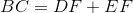Statement 2: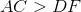and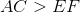BOTH statements TOGETHER are insufficient to answer the question.

Statement 1 ALONE is sufficient to answer the question, but Statement 2 ALONE is NOT sufficient to answer the question.

Statement 2 ALONE is sufficient to answer the question, but Statement 1 ALONE is NOT sufficient to answer the question.

BOTH statements TOGETHER are sufficient to answer the question, but NEITHER statement ALONE is sufficient to answer the question.

EITHER statement ALONE is sufficient to answer the question.

Statement 1 ALONE is sufficient to answer the question, but Statement 2 ALONE is NOT sufficient to answer the question.

Explanation:

Assume Statement 1 alone.is equilateral, so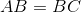. Also, by the Triangle Inequality, the sum of the lengths of two sides of a triangle must exceed the third, so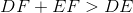. From Statement 1,, so by substitution,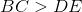, and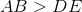.

Statement 2 alone provides insufficient information. For example, assumeis an equilateral triangle with sidelength 9. Ifis an equilateral triangle with sidelength 8, the conditions of the statement hold, and. However, ifis a right triangle in which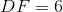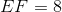, and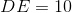, the conditions of the statement still hold, but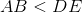.

### Example Question #13 : Equilateral Triangles

Given equilateral trianglesand, which, if either, is longer,or?

Statement 1: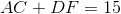Statement 2: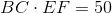Statement 2 ALONE is sufficient to answer the question, but Statement 1 ALONE is NOT sufficient to answer the question.

BOTH statements TOGETHER are insufficient to answer the question.

EITHER statement ALONE is sufficient to answer the question.

Statement 1 ALONE is sufficient to answer the question, but Statement 2 ALONE is NOT sufficient to answer the question.

BOTH statements TOGETHER are sufficient to answer the question, but NEITHER statement ALONE is sufficient to answer the question.

BOTH statements TOGETHER are insufficient to answer the question.

Explanation:

All sides of an equilateral triangle have the same measure, so we can letbe the common sidelength of, and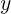be that of.

Statement 1 can be rewritten as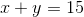; Statement 2 can be rewritten as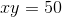. The equivalent question is whether we can determine which, if either, is greater,or. The two statements together are insufficient to answer the question, however; 5 and 10 have sum 15 and product 50, but we cannot determine without further information whetherand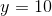, or vice versa. Therefore, we do not know for sure whether a side ofis longer than a side of- specifically, which oforis longer.

### Example Question #14 : Equilateral Triangles

Given equilateral trianglesand, which, if either, is longer,or?

Statement 1: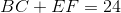Statement 2: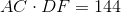Statement 1 ALONE is sufficient to answer the question, but Statement 2 ALONE is NOT sufficient to answer the question.

EITHER statement ALONE is sufficient to answer the question.

BOTH statements TOGETHER are sufficient to answer the question, but NEITHER statement ALONE is sufficient to answer the question.

BOTH statements TOGETHER are insufficient to answer the question.

Statement 2 ALONE is sufficient to answer the question, but Statement 1 ALONE is NOT sufficient to answer the question.

BOTH statements TOGETHER are sufficient to answer the question, but NEITHER statement ALONE is sufficient to answer the question.

Explanation:

All sides of an equilateral triangle have the same measure, so we can letbe the common sidelength of, andbe that of.

Statement 1 can be rewritten as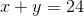; Statement 2 can be rewritten as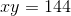. The equivalent question is whether we can determine which, if either, is greater,or.

Statement 1 alone yields insufficient information; for example, the two numbers added together could be 10 and 14, but it is impossible to determine whetheroris the greater of the two. Statement 2 alone is also insufficient, for a similar reason; for example, the two numbers could be 9 and 16, but again, eitherorcould be the greater.

Now assume both statements. The only two numbers that can be added to yield a sum of 24 and multiplied to yield a product of 144 are 12 and 12; therefore,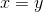, andandhave the same sidelengths. Specifically,andhave the same length.

2 Next →

Tired of practice problems?

Try live online GMAT prep today.